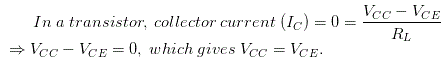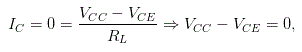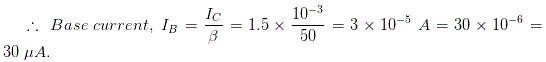# MCQs on Analog Electronics

##### Page 31 of 33. Go to page 1 2 3 4 5 6 7 8 9 10 11 12 13 14 15 16 17 18 19 20 21 22 23 24 25 26 27 28 29 30 31 32 33
01․ Three difference Q points are shown on a load line. The upper Q point represents the
minimum current gain.
intermediate current gain.
maximum current gain.
cutoff point.

The upper Q point represents the high output current and that means the Q point represents the maximum current gain.

02․ If a transistor operates at the middle of the load line, a decrease in the base resistance will move the Q point
down.
up.
nowhere.

Whenever the base resistance decreases, the base current increases according to the base resistance decrements. We know that the base current is directly proportional to the output collector current. That small change in the base current gives the big increment in the output current. It means that the Q point move up from the previous level.

03․ If a transistor operates at the middle of the load line, a decrease in the current gain will move the Q point
down.
up.
nowhere.

Reduction in current gain leads to reduce the output current because both are proportional to each other. Obviously the Q point goes down, if the output current decreases.

04․ If the base supply voltage increase, the Q point moves
down.
up.
nowhere.

According to the ohms law, the voltage is directly proportional to current. Hence, the base current also increases due to the increases in base voltage. This small change in the base current gives the big increment in the output current. It means that the Q point move up from the previous level.

05․ Suppose the base resistor is open. The Q point will be
in the middle of the load line.
at the upper end of the load line.
at the lower end of the load line.

If the base resistance is open, there is no path to flow the current from the source to the base of the transistor. Hence, the base current is 0 Amps. It leads to the output current as very much as lower but there can be a small leakage current due to the collector diode. So, the Q point goes lower end of the load line.

06․ If the base supply voltage is disconnected, the collector-emitter voltage will equal
0 V.
6 V.
10.5 V.
collector supply voltage.

In a transistor, if the base supply voltage is zero then the base current is also zero. We know that the base current and collector current are directly proportional. So, the collector current is also zero.Here, the VCC is collector supply voltage and the VCE is collector emitter voltage.

07․ If the base resistor has zero resistance, the transistor will probably be
saturated.
in cutoff.
destroyed.
none of the above.

If the base resistance is zero, then the base current is very high. Since, we know that the base current is directly proportional to the collector current with the multiplying factor of current gain. So, the value of collector current is enormously getting high. In this time, the transistor operates in saturation region and finally it is being destroyed.

08․ If the collector resistor opens in a base-biased circuit, the load line will become
horizontal.
vertical.
useless.
flat.

When the collector resistor opens, then the collector current is zero. If the collector currentwhich gives VCC = VCE. Hence, the load line permanently reaches the horizontal as shown in below characteristics graph of a transistor. That means the transistor is in cut-off region.

09․ The collector current is 1.5 mA. If the current gain is 50, the base current is
3 µA.
30 µA.
150 µA.
3 mA.

We know that the collector current IC = βIB.10․ When the Q point moves along the load line, VCE decreases when the collector current
decreases.
stays the same.
increases.
does none of the above.

The below characteristics curve of transistor shows that when the Q point moves along the load line, VCE decreases when the collector current increases.

<<<2930313233>>>